# 6.13. Exercises¶

1. Use the drawsquare function we wrote in this chapter in a program to draw the image shown below. Assume each side is 20 units. (Hint: notice that the turtle has already moved away from the ending point of the last square when the program ends.)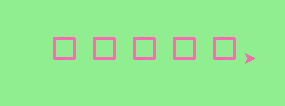1. Write a program to draw this. Assume the innermost square is 20 units per side, and each successive square is 20 units bigger, per side, than the one inside it.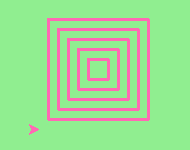1. Write a non-fruitful function `drawPoly(someturtle, somesides, somesize)` which makes a turtle draw a regular polygon. When called with `drawPoly(tess, 8, 50)`, it will draw a shape like this: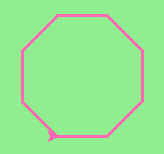1. Draw this pretty pattern.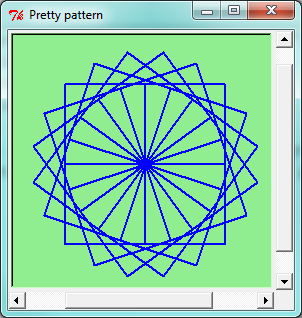1. The two spirals in this picture differ only by the turn angle. Draw both.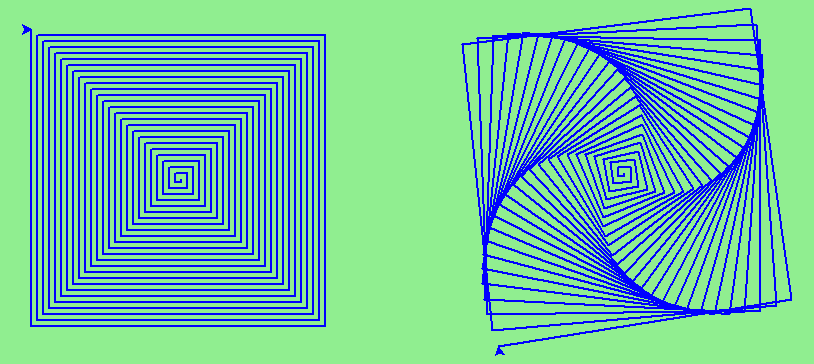1. Write a non-fruitful function `drawEquitriangle(someturtle, somesize)` which calls `drawPoly` from the previous question to have its turtle draw a equilateral triangle.

1. Write a fruitful function `sumTo(n)` that returns the sum of all integer numbers up to and including n. So `sumTo(10)` would be `1+2+3...+10` which would return the value 55. Use the equation (n * (n + 1)) / 2.

1. Write a function areaOfCircle(r) which returns the area of a circle of radius r. Make sure you use the math module in your solution.

1. Write a non-fruitful function to draw a five pointed star, where the length of each side is 100 units.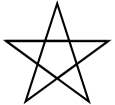1. Extend your program above. Draw five stars, but between each, pick up the pen, move forward by 350 units, turn right by 144, put the pen down, and draw the next star. You’ll get something like this (note that you will need to move to the left before drawing your first star in order to fit everything in the window):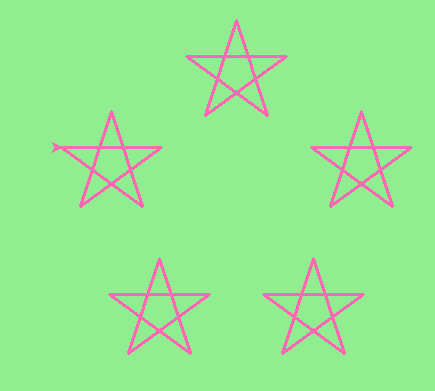What would it look like if you didn’t pick up the pen?

1. Extend the star function to draw an n pointed star. (Hint: n must be an odd number greater or equal to 3).

1. Write a function called drawSprite that will draw a sprite. The function will need parameters for the turtle, the number of legs, and the length of the legs. Invoke the function to create a sprite with 15 legs of length 120.

1. Rewrite the function `sumTo(n)` that returns the sum of all integer numbers up to and including n. This time use the accumulator pattern.

1. Write a function called `mySqrt` that will approximate the square root of a number, call it n, by using Newton’s algorithm. Newton’s approach is an iterative guessing algorithm where the initial guess is n/2 and each subsequent guess is computed using the formula: newguess = (1/2) * (oldguess + (n/oldguess)).
1. Write a function called `myPi` that will return an approximation of PI (3.14159…). Use the Leibniz approximation.
1. There was a whole program in A Turtle Bar Chart to create a bar chart with specific data. Creating a bar chart is a useful idea in general. Write a non-fruitful function called barChart, that takes the numeric list of data as a parameter, and draws the bar chart. Write a full program calling this function. The current version of the `drawBar` function unfortuately draws the top of the bar through the bottom of the label. A nice elaboration is to make the label appear completely above the top line. To keep the spacing consistent you might pass an extra parameter to `drawBar` for the distance to move up. For the `barChart` function make that parameter be some small fraction of `maxheight+border`. The fill action makes this modification particularly tricky: You will want to move past the top of the bar and write before or after drawing and filling the bar.